Contradiction between FitzGerald-Lorentz Length Contraction and Time Dilation: A GPS-Compatible Lorentz Transformation | OMICS International
Journal of Biosensors & Bioelectronics

Like us on:

# Contradiction between FitzGerald-Lorentz Length Contraction and Time Dilation: A GPS-Compatible Lorentz Transformation

Buenker RJ*

Department of C-Mathematics and Science, University of Wuppertal, Wuppertal, Germany

*Corresponding Author:
Robert J Buenker
Department of C-Mathematics and Science
University of Wuppertal, Gaussstr 20, D-42119 Wuppertal, Germany
Tel: +49-202-439-2774
E-mail: [email protected]

Received date: July 27, 2016; Accepted date: July 29, 2016; Published date: August 09, 2016

Citation: Buenker RJ (2016) Contradiction between FitzGerald-Lorentz Length Contraction and Time Dilation: A GPS-Compatible Lorentz Transformation. J Biosens Bioelectron 7:215. doi:10.4172/2155-6210.1000215

Copyright: © 2016 Buenker RJ. This is an open-access article distributed under the terms of the Creative Commons Attribution License, which permits unrestricted use, distribution, and reproduction in any medium, provided the original author and source are credited.

Visit for more related articles at Journal of Biosensors & Bioelectronics

#### Abstract

Recent work has shown that the underlying assumptions of the Global Positioning System (GPS) are incompatible with a number of conclusions of conventional relativity theory, including Fitzgerald-Lorentz length contraction (FLC) and the supposed ambiguity in the relative rates of clocks in motion. The challenge is therefore to amend relativity theory so as to bring it into full consistency with the experience of the GPS methodology. This goal must be accomplished while at the same time avoiding conflict with other predictions of relativity theory that have received ample experimental confirmation over the years. It is shown that this can be achieved by eliminating an undeclared assumption in Einstein’s derivation of the Lorentz transformation (LT) and replacing it with the GPS axiom of the strict proportionality of clock rates in different rest frames. The standard relativistic velocity transformation still retains its validity thereby. However, the relationships between respective measured values of observers in relative motion for the same quantity are determined in the revised theory with the aid of a simple scaling procedure in each case.

#### Keywords

Time dilation; Uniform scaling; Relativistic velocity transformation (RVT); Global positioning system (GPS); Lorentz transformation (LT); Universal time-dilation law (UTDL); Alternative lorentz transformation (GPS-LT)

#### Introduction

One of the cornerstones of the Global Positioning System (GPS) methodology is the assumption that clocks on orbiting satellites and on the earth’s surface have rates which are strictly proportional to one another at all times [1,2]. It has been shown  that the second postulate (light-speed constancy) of Einstein’s special theory of relativity (STR ) requires that the dimensions of objects must accordingly have increased uniformly in all directions as a result of being shot into orbit from their initial position on the earth’s surface. This result stands in direct contradiction with the well-known STR prediction of Fitzgerald- Lorentz length contraction (FLC). It is also inconsistent with the equivalence principle  since the latter predicts that the lengths of objects remain constant as they are accelerated, just as when they change their position in a gravitational field.

Yet the very success of the GPS methodology in measuring distances serves as a clear verification of the relativistic assumptions upon which it is based. For example, it rests squarely on the two postulates employed by Einstein  in his original work to define STR. It also makes direct use of his conclusion that clocks must slow down as a result of being accelerated. In the following discussion it will be shown that there is nonetheless a problem with the derivation of the Lorentz transformation (LT). This eventuality is directly responsible for the theory’s false predictions of anisotropic length contraction (FLC) and the subjectivity of measurement, whereby, for example, two clocks in relative motion can supposedly each be running slower than the other.

Critical assumptions in the existing theory (STR)

The derivation of Fitzgerald-Lorentz length contraction (FLC) rests on the following equation of the generalized version of the LT :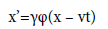(1)

in which x and x’ are the respective measurements of two observers for the position of an object on the axis parallel to their relative velocity v. The measurement is made at time t for the “stationary” observer [γ =(1 – v2/c2)-0.5 and c is the speed of light (299792458 ms-1), which is referred to as V in the original notation]. Einstein stated without further discussion that φ is an “as yet unknown function of the speed v”. He then went on to show that the only acceptable value for φ under this restrictive definition is unity.

However, any other value for φ in equation (1) is also consistent with Einstein’s postulate of the constancy of the speed of light in free space. The corresponding equation for the temporal variables is:

t’=γφ(t – vxc-2) (2)

Where again the value of φ=1 leads to the corresponding LT relation. The STR formula for time dilation (t’=γ-1t) results by setting φ=1 and x=vt for the coordinates of the moving observer in equation (2). Both the FLC and time dilation effects are predicted to be symmetric with respect to interchanging the coordinates of the two observers. However, the transverse Doppler experiments with high-speed rotors  and the GPS methodology both demonstrate that an accelerated clock runs slower than its stationary counterpart, in clear violation of the STR prediction of complete ambiguity.

Division of equation (2) by equation (1) leads to the corresponding equation in the relativistic velocity transformation (RVT) for the respective x-components (ux and ux’) of the velocity which Einstein derived in his original work . The key point is that the choice of φ is immaterial in arriving at the latter result because this function is eliminated as a result of the division. The same holds true for the relationship between the respective transverse (y,z) velocity components, so that the RVT equations are obtained as: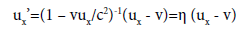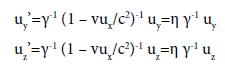(3)

In these equations ux= x/t, uy= y/t and uz= z/t in the stationary rest frame, with analogous equations for ux’, uy’ and uz’ in the moving frame [η=(1–vux/c2)-1]. It is essential that the RVT be incorporated in any revised theory because its predictions have been confirmed experimentally (aberration of starlight from the zenith and the Fresnel light drag formula , as well as Thomas spin precession .

It is important to note that Einstein had two fundamentally different positions on the nature of time dilation in his original work . On the one hand, it was concluded on the basis of the LT that the effect was symmetric, as already discussed, but on the other, it was claimed that a clock at the Equator must run slightly slower than one at either of the Poles because of the increase in the speed of rotation about the earth’s axis with decreasing latitude. The point that needs to be emphasized is that the latter prediction is based on the conclusion that it is possible to distinguish between rest frames and therefore that there is no ambiguity as to which clock runs slower in the above example. The exact opposite position is taken with regard to the prediction of time dilation based on the LT. Sherwin  has argued this distinction arises because the LT is only applicable to uniformly moving (inertial) systems and therefore does not apply for clocks at rest on the earth’s surface.

Although Einstein did not give an explicit formula for the way in which clock rates vary with latitude on the earth’s surface , it is a simple matter to derive one based on the above prediction. Accordingly, the elapsed time τ measured for a given event is inversely proportional to γ (v0), where v0 is the speed of the clock relative to the rest frame of the earth’s center of mass (ECM). The corresponding equation for the elapsed times τ1 and τ2 of two clocks located at arbitrary latitudes is therefore,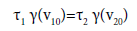(4)

where v10 and v20 are their respective speeds relative to the ECM. This formula is also applicable to high-speed rotors [6,9], in which case the corresponding axis serves as the unique reference frame from which v10 and v20 for the two clocks are defined. It is clearly possible that the clocks being compared might have different reference frames, so in that case one has to know the relationship between the rates of clocks that are at rest in the respective reference frames to apply equation (4). With this complication in mind, it is possible to look upon equation (4) as a universal time-dilation law (UTDL) in the absence of gravitational effects. In the present context the most important aspect of the law is that it is consistent with the strict proportionality of clock rates assumed in the GPS methodology [1,2]. It is also clear from equation (4) that the ratio of clock rates can take on any value, greater or smaller than unity, unlike the case for the corresponding prediction from the LT.

Outline of the modified theory

Based on the discussion in the preceding section, it is surprisingly easy to revise the existing version of STR to bring it into full consistency with the GPS methodology. The primary objective is to combine the UTDL of equation (4) with the RVT. This can be accomplished by employing a different assumption to specify the function φ in equations (1-2) than was used in Einstein's original derivation of the LT . Consistent with GPS, one must simply assume that elapsed times measured by the two observers in motion are strictly proportional to one another: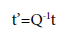(5)

where the value of the proportionality constant is determined either by experiment or with the application of the UTDL. The value of φ can then be obtained as a function of Q by combining equation (5) with equation (2). Alternatively, one can multiply the three equations of the RVT in equation (3) with t’ on the left and with Q-1t on the right in each case. The other three equations [in addition to equation (5)] of the resulting space-time transformation, which replaces the LT in the modified theory, are thus: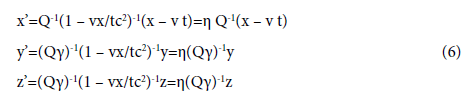Accordingly, the value of φ in equations (1-2) is:

φ=η(Qγ)-1 (7)

which is seen to be a function of both ux=x/t and the relative speed v, not simply the latter as Einstein assumed . Note that η is already present in the RVT, so the idea that φ must only be a function of v in the relativistic modification of the Galilean transformation could already have been questioned on this basis.

The proportionality constant Q appears explicitly in all four equations of the modified LT, but not in the RVT. The latter fact is consistent with Einstein’s second postulate since it shows that the measured values of particle speeds are completely independent of the rates of the (proper) clocks used to measure them. It also points up a conceptually simpler means of applying the modified theory to the determination of distances: first establish the mutual value measured by the two observers of the speed u of a particle as it moves between fixed points in space; then compute the values of the corresponding distance between the same points for each of them by multiplying their respective elapsed times for the particle’s journey with u. In that way one sees clearly that the observer with the slower clock will measure a smaller value for the corresponding distance. This result is sometimes claimed in texts [10,11] to be evidence for length contraction in the “moving” rest frame, whereas quite the opposite is true; the reason the latter obtains a smaller value for the distance is because the length of his measuring device (unit of distance) is larger than in the corresponding “stationary” rest frame. It also is often overlooked that the so-called contraction is independent of the orientation of the measuring device to the object of the measurement, which is in any event contrary to the prediction of the FLC.

The latter discussion calls attention to a fundamental difference in the way the two versions of relativity theory go about making their predictions. The basic procedure in STR is to use a linear transformation connecting the measured values for the same event in two rest frames in (uniform) relative motion. The same objective is achieved in the present revised theory with a separate scaling procedure for each physical property. The proportionality factor Q in equation (5) plays a central role in all such determinations. It is convenient to look upon the latter as a “conversion factor” that defines a change in units between the two rest frames. Exactly the same conversion factor (Q) is used in relating distances between fixed points. This result follows directly from Einstein’s second postulate, as already mentioned above.

The revised theory assumes that the measurement process is perfectly objective, however, so this means that there is a reciprocal relationship between the conversion factors employed in the two rest frames. This is easily seen by inverting equation (5), from which one obtains:

t=Qt’=Q’-1t’ (8)

Where,

Q’ is the “reverse” scale factor to be used in converting in the opposite direction between the two rest frames (QQ’=1). By using the reciprocal relation between Q and Q', one guarantees that Einstein's other postulate of relativity , the relativity principle (RP) is also satisfied . Conversion factors cannot normally be used in STR because a symmetric relationship is assumed between the measured values in this theory. An attempt to do so would mean that one uses the same conversion factor in both directions, which obviously leads to nonsensical results.

In order to have a suitably general range of applicability, the revised theory also needs to clearly specify how the scaling is to be carried out for other properties. One is helped thereby by the experimental fact that the inertial mass mI of an object such as an electron increases upon acceleration by the same factor as do the lifetimes of meta-stable particles. One can safely conclude thereby that the units of inertial mass and time vary in strict proportion to one another, which means that Q is also the appropriate conversion factor in this case. Because of Einstein’s E=mc2 relation, it follows that the energy E scales in the same manner since c and relative speeds in general are the same for observers in different rest frames. Indeed, once the conversion factors for the three units of time, space and inertial mass have been defined, it is a simple manner to determine the corresponding results for any other physical property. Thus, force scales as Q0, the same as relative velocities, and angular momentum scales as Q2. Also, frequencies must scale as Q-1 because of their inverse relationship to periods. These conclusions are straightforward because of the overriding assumption in the revised theory that the measurement process is strictly objective, something that is categorically denied by STR. Since Planck’s constant has units of angular momentum, it follows that it must vary as Q2, a prediction of the revised theory that can conceivably be verified on the basis of measurements of the photoelectric effect.

In the above discussion it is imperative that one draw a clear distinction between comparing velocities of the same particle relative to two different observers that are in relative motion on the one hand, and velocities between two fixed points relative to the same two observers. In the first case, one is actually comparing two fundamentally different quantities, i.e., the velocity of the object with respect to observer A (vA) and the velocity of the same particle with respect to observer B (vB). One needs a linear transformation in the form of the RVT to obtain the desired information in this case, and the typical finding is that vA ≠ vB (this is also true for light pulses since even though the speed is the same for both observers, the direction is not). In the other case, both observers are measuring exactly the same quantity, namely the velocity of a given particle between two fixed points, and the RVT shows explicitly that the result must be the same for both observers . This in turn is consistent with the assumption that relative speeds scale as Q0.

The transverse Doppler effect provides a good illustration of how linear transformations can be avoided entirely in the revised theory for an application where they are indispensable in the corresponding STR treatment. In the latter theory it is assumed that the light frequency and wavelength in each rest frame form separate four-vectors that are related by the LT. On this basis one concludes  that the emitted frequency will be γ (v) times smaller (red-shifted) when the light waves arrive at the detector in a stationary laboratory than is the case for an observer co-moving with the source (v is the relative speed of the two rest frames). The prediction is the same independent of whether the detector or the light source undergoes greater acceleration, in contrast to what is found experimentally using high-speed rotors [6,9]. The present version of the theory simply makes use of the UTDL of equation (4) to predict that the more accelerated clock relative to the rotor axis will run slower and by what factor [Q in equations (5,8)]. As a consequence, a blue shift is expected if the detector/absorber is located farther out on the rotor than the light source, whereas a red shift is predicted for the opposite placement, in quantitative agreement with observation. Neither the RVT of equation (3) nor the alternative Lorentz transformation (GPS-LT) of equation (6) is required to obtain this result in the present version of the theory.

A similar situation exists for energy E and momentum p. In the STR formulation these quantities form equivalent four-vectors in each rest frame which satisfy the following invariance relation: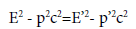(9)

For the special case in which one of the rest frames is co-moving with an accelerated particle (p’=0) and the other is the rest frame of the laboratory observer, equation (9) reduces to: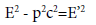(10)

Where,

E’ is thus the rest (or proper) energy of the particle. This result has been verified to high accuracy experimentally, but it also can be obtained within the framework of the present revised theory with a scaling relation analogous to equation (5), namely:

E’=Q-1 E (11)

for the special case with Q=γ. Squaring equation (11) leads directly to equation (10) with the help of the definition of momentum as p=mIv=Evc-2 and E=mIc2.

As with other applications, however, there is also a clear distinction between the predictions of the two theories in this case as well. The value of the conversion factor of Q=γ is too restrictive. In the present revised theory Q is determined by the UTDL of equation (4) because energy scales in exactly the same way as time and distance. Thus any value for Q is possible depending on the respective speeds of the particle and observer with respect to the reference frame implied in the latter formula. It is not true, for example, that an observer co-moving with the accelerated particles would find that particles at rest in the laboratory would have γE’ for their energy. The conversion factor in this case is the reciprocal (Q’=Q-1-1) of that used by the observer at rest in the laboratory and thus, in accord with equation (8), a value of γ-1E’ must be obtained in this case.

The key point in the above discussion is that the revised theory subscribes to the unequivocal objectivity of measurement, whereas quite the opposite is true in STR because of its fundamental assumption that inertial systems are completely indistinguishable. It is also assumed in the revised theory that the laws of physics are the same in each inertial system (Einstein's first postulate of relativity), but that the physical units on which they are based may, and generally do, differ. This interpretation is perfectly consistent with Galileo’s original definition of the RP and, just as importantly, is essential in order to remain consistent with experimental observations of clock rates and the fundamental assumptions of the GPS methodology.

#### Conclusion

The Fitzgerald-Lorentz length contraction effect (FLC) holds that the dimensions of a moving object decrease by varying amounts depending on its orientation relative to a stationary observer. It has been shown in previous work that quite the opposite result (isotropic expansion) must occur for objects located on satellites if one bases the analysis on the standard assumptions employed in the GPS methodology. This includes first and foremost the conclusion that clock rates on orbiting satellites are strictly proportional to those on the earth’s surface, which runs contrary to the assertion of STR that space and time are not distinct quantities. Examination of Einstein’s original work on relativity theory  shows that both the space-time mixing and FLC are based on an unwarranted assumption, namely that a certain function involved in the derivation of the Lorentz transformation (LT) can only depend on the relative speed v of two inertial rest frames. By replacing this assumption with the GPS clock-rate proportionality, it turns out that it is still possible to satisfy Einstein’s two postulates of relativity theory. In particular, Einstein’s relativistic velocity transformation (RVT) is not affected thereby.

Perhaps the most important characteristic of the revised theory is its adherence to a strictly objective view of the measurement process. This is in stark contrast to what is claimed in the STR version of the theory, where one assumes that two clocks can both be running slower than the other when they are in relative motion. In the present revised theory it is always possible in principle to know which clock rate is faster, which distance is longer and which mass is greater. One only needs to know the appropriate scale factor for a given property, and this information can be obtained from the theory in a relatively simple manner with the aid of the UTDL of equation (4). The proportionality factor for lengths and energies is exactly the same as for elapsed times. The corresponding values for other mechanical properties can be obtained by knowing their composition in terms of the latter three quantities. This means that relative velocities between fixed points always have the same value for different observers , for example, since the scaling of time and distance cancels in this case. The same holds true for forces by virtue of the equal scale factors for energy and distance.

The revised theory can perhaps be most simply described in terms of the variation of standard units with changes in the state of motion of the object and the observer. One doesn’t notice any change in units on the basis of in situ measurements of objects in one’s own rest frame because of the uniformity in the scaling. This position is perfectly consistent with Galileo’s statement of the RP, where the observers were isolated in the hold of a ship on a calm sea. The laws of physics remain the same when the system of units is changed as long as this is done in a consistent manner. This happens automatically when an observer is accelerated. He only needs to be aware that changes in standard units have occurred when he wishes to compare his measured values with someone else’s in another rest frame. There is a separate scaling of physical units for changes in gravitational potential, as discussed elsewhere , but the principles involved are closely related to the “kinetic” type of scaling discussed in the present work. Finally, the assumption of strict proportionality of clock rates is also consistent with the principle of absolute remote simultaneity of events that has long been denied by STR because of its treatment of time dilation. This principle is also essential for the successful operation of the GPS technology and is incorporated in the revised theory by virtue of equation (5).

#### References

Select your language of interest to view the total content in your interested language

### Article Usage

• Total views: 13054
• [From(publication date):
September-2016 - Dec 13, 2019]
• Breakdown by view type
• HTML page views : 12929Can't read the image? click here to refresh